# NCERT Solutions for Chapter 5 Understanding Elementary Shapes Class 6 Maths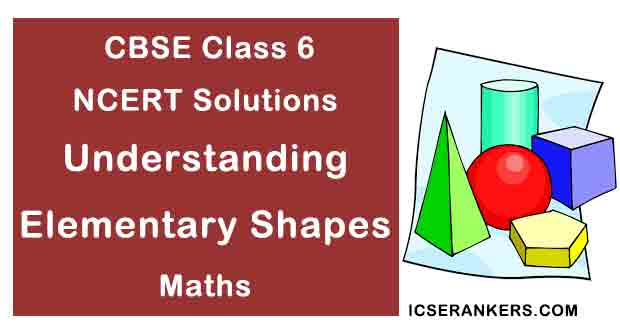### Understanding Elementary Shapes Questions and Answers

 Chapter Name Understanding Elementary Shapes NCERT Solutions Class CBSE Class 6 Textbook Name Maths Related Readings NCERT Solutions for Class 6NCERT Solutions for Class 6 Maths Summary of Understanding Elementary Shapes

Exercise 5.1

Question 1: What is the disadvantage in comparing line segments by mere observation?

Solution

There may be chance of error due to improper viewing.

Question 2: Why is it better to use a divider than a ruler, while measuring the length of a line segment?

Solution

It is better to use a divider than a ruler, because the thickness of the ruler may cause difficulties in reading off her length. However divider gives up accurate measurement.

Question 3: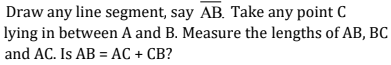[Note: If A, B, C are any three points on a line, such that AC + CB = AB, then we can be sure that C lies between A and B.]

Solution

Yes.AB = 6.5 cm, AC = 3cm, CB = 3.5 cm

AC + CB = 3 cm + 3.5 cm = 6.5 cm = AB

Question 4: If A, B, C are three points on a line such that AB = 5 cm, BC = 3cm and AC = 8 cm, which one of them lies between the other two?

Solution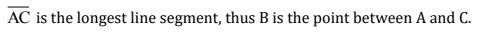Question 5: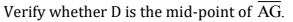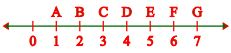Solution

AD = 3 units, DG = 3 units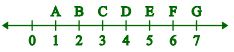Thus, D is the mid-point.

Question 6: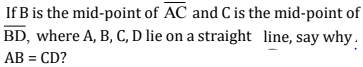Solution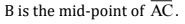∴   AB=BC       ....(i)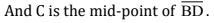∴  BC=CD        ....(ii)

From equation (i) and (ii), we get

AB = CD

Question 7: Draw five triangles and measure their sides. Check in each case, of the sum of the lengths of any two sides is always less than the third side.

Solution

Yes, sum of two sides of a triangle is always greater than the third side.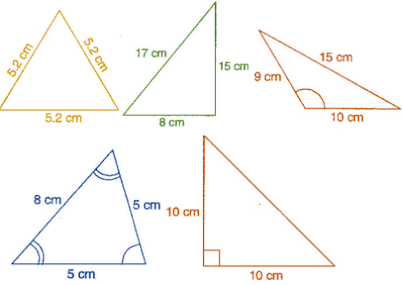Exercise 5.2

Question 1: What fraction of a clockwise revolution does the hour hand of a clock turn through, when it goes from

(a) 3 to 9

(b) 4 to 7

(c) 7 to 10

(d) 12 to 9

(e) 1 to 10

(f) 6 to 3

Solution

(a) 1/2 or two right angles

(b) 1/4 or one right angle

(c) 1/4 or one right angle

(d) 3/4 or three right angles.

(e) 3/4 or three right angles.

(f) 3/4 or three right angles.

Question 2: Where will the hand of a clock stop if it:

(a) starts at 12 and make 1/2 of a revolution, clockwise?

(b) starts at 2 and makes 1/2 of a revolution, clockwise?

(c) starts at 5 and makes 1/4 of a revolution, clockwise?

(d) starts at 5 and makes 3/4 of a revolution, clockwise?

Solution

(a) At 6

(b) At 8

(c) At 8

(d) At 2

Question 3: Which direction will you face if you start facing:

(a) East and make 1/2 of a revolution clockwise?

(b) East and make 1 ½  of a revolution clockwise?

(c) West and makes 3/4 of a revolution, clockwise?

(d) South and make one full revolution?

(Should we specify clockwise or anti-clockwise for this last question? Why not?)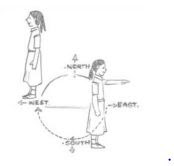Solution

(a) West

(b) West

(c) North

(d) South

(For answer (d), it is immaterial whether we turn clockwise or anticlockwise, because one full revolution will bring us back to the original position)

Question 4: What part of a revolution have you turned through if you stand facing:

(a) East and turn clockwise to face north?

(b) South and turn clockwise to face east?

(c) West and turn clockwise to face east?

Solution

(a) 3/4

(b) 3/4

(c) 1/2

Question 5: Find the number of right angles turned through by the hour hand of a clock when it goes from:

(a) 3 to 6

(b) 2 to 8

(c) 5 to 11

(d) 10 to 1

(e) 12 to 9

(f) 12 to 6

Solution

(a) One right angle

(b) Two right angles

(c) Two right angles

(d) One right angle

(e) Three right angles

(f) Two right angles

Question 6: How many right angles do you make if you start facing:

(a) South and turn clockwise to west?

(b) North and turn anti-clockwise to east?

(c) West and turn to west?

(d) South and turn to north?

Solution

(a) One right angle

(b) Three right angles

(c) Four right angles

(d) Two right angles

Question 7: Where will the hour hand of a clock stop if it starts:

(a) from 6 and turns through 1 right angle?

(b) from 8 and turns through 2 right angles?

(c) from 10 and turns through 3 right angles?

(d) from 7 and turns through 2 straight angles?

Solution

(a) At 9

(b) At 2

(c) At 7

(d) At 7

Exercise 5.3

Question 1: Match the following:

 Column I Column II (i) Straight angle (a) less than one-fourth a revolution (ii) Right angle (b) more than half a revolution (iii) Acute angle (c) half of a revolution (iv) Obtuse angle (d) one-fourth a revolution (v) Reflex angle (e) between 1/4 and 1/2 of a revolution (f) one complete revolution

Solution

 Column I Column II (i) Straight angle (c) half of a revolution (ii) Right angle (d) one-fourth a revolution (iii) Acute angle (a) less than one-fourth a revolution (iv) Obtuse angle (e) between 1/4 and 1/2 of a revolution (v) Reflex angle (b) more than half a revolution

Question 2: Classify each one of the following angles as right, straight, acute, obtuse or reflex: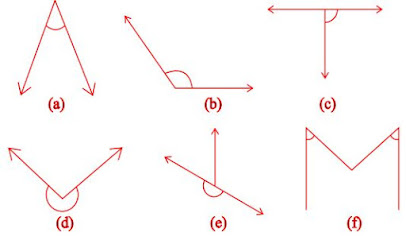Solution

(a) Acute angle

(b) Obtuse angle

(c) Right angle

(d) Reflex angle

(e) Straight angle

(f) Acute angle

Exercise 5.4

Question 1: What is the measure of

(i) a right angle?

(ii) a straight angle?

Solution

(i) 90°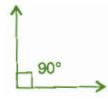(ii) 180°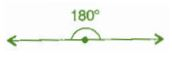Question 2: Say True or False:

(a) The measure of an acute angle < 90° .

(b) The measure of an obtuse angle < 90° .

(c) The measure of a reflex angle > 180° .

(d) The measure of on complete revolution = 360° .

(e) If mA = 53° and mB = 35° , then mA  > mB.

Solution

(a) True

(b) False

(c) True

(d) True

(e) True

Question 3: Write down the measure of:

(a) some acute angles

(b) some obtuse angles

(give at least two examples of each)

Solution

(a) 35° ,20°

(b) 110° ,135°

Question 4: Measure the angles given below, using the protractor and write down the measure: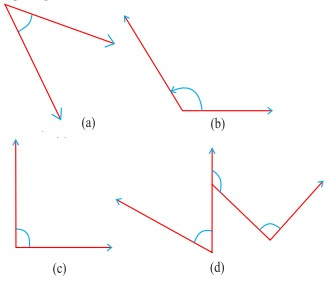Solution

(a) 40°

(b) 130°

(c) 90°

(d) 60°

Question 5: Which angle has a large measure? First estimate and then measure:

Measure of angle A =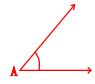Measure of angle B =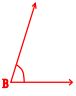Solution

∠B has larger measure.

∠A =40° and ∠B = 65°

Question 6: From these two angles which has larger measure? Estimate and then confirm by measuring them:Solution

Second  angle has larger measure.

Question 7: Fill in the blanks with acute, obtuse, right or straight:

(a) An angle whose measure is less than that of a right angle is ________.

(b) An angle whose measure is greater than that of a right angle is _______.

(c) An angle whose measure is the sum of the measures of two right angles is ________.

(d) When the sum of the measures of two angles is that of a right angle, then each one of them is _________.

(e) When the sum of the measures of two angles is that of a straight angle and if one of them is acute then the other should be _________.

Solution

(a) acute angle

(b) obtuse angle

(c) straight angle

(d) acute angle

(e) obtuse angle

Question 8: Find the measure of the angle shown in each figure. (First estimate with your eyes and then find the actual measure with a protractor).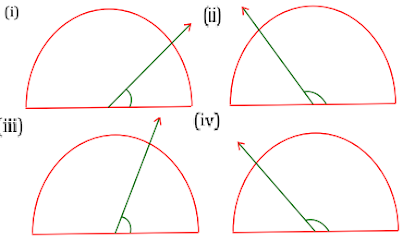Solution

(i) 30°

(ii) 120°

(iii) 60°

(iv) 150°

Question 9: Find the angle measure between the hands of the clock in each figure: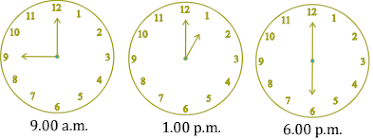Solution

(i) 90° (Right angle)

(ii) 30° (Acute angle)

(iii) 180° (Straight angle)

Question 10: Investigate:

In the given figure, the angle measure 30° . Look at the same figure through a magnifying glass. Does the angle becomes larger? Does the size of the angle change?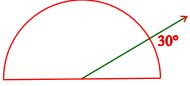Solution

No, the measure of angle will be same.

Question 11: Measure and classify each angle: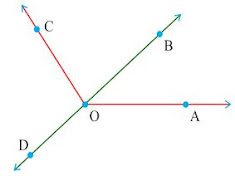Angle Measure Type ∠AOB ∠AOC ∠BOC ∠DOC ∠DOA ∠DOB

Solution

 Angle Measure Type ∠AOB 40° Acute ∠AOC 130° Obtuse ∠BOC 90° Right ∠DOC 90° Right ∠DOA 140° Obtuse ∠DOB 180° Straight

Exercise 5.5

Question 1: Which of the following are models for perpendicular lines:

(a) The adjacent edges of a table top.

(b) The lines of a railway track.

(c) The line segments forming the letter ‘L’.

(d) The letter V.

Solution

(a) Perpendicular

(b) Not perpendicular

(c) Perpendicular

(d) Not perpendicular

Question 2: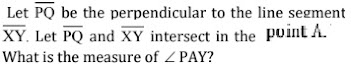Solution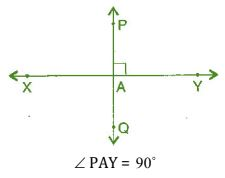Question 3: There are two “set-squares” in your box. What are the measures of the angles that are formed at their corners? Do they have any angle measure that is common?

Solution

One set-square has 45° ,90° ,45° and other set-square has 60° ,90° ,30° . They have 90° as common angle.

Question 4: Study the diagram. The line l is perpendicular to line m.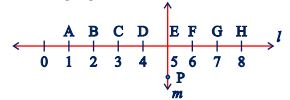(a) Is CE = EG?

(b) Does PE bisect CG?

(c) Identify any two line segments for which PE is the perpendicular bisector.

(d) Are these true?  (i) AC > FG  (ii) CD = GH  (iii) BC < EH

Solution

(a) Yes, both measure 2 units.

(b) Yes, because CE = EG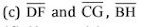(d) (i) True, (ii) True, (iii) True

Exercise 5.6

Question 1: Name the types of following triangles:

(a) Triangle with lengths of sides 7 cm, 8 cm and 9 cm.

(b) ABC with AB = 8.7 cm, AC = 7 cm and BC = 6 cm.

(c) PQR such that PQ = QR = PR = 5 cm.

(d) DEF with mD = 90°

(e) XYZ with mY = 90° and XY = YZ

(f) LMN with mL = 30° , mM = 70° and mN = 80° .

Solution

(a) Scalene triangle

(b) Scalene triangle

(c) Equilateral triangle

(d) Right-angled triangle

(e) Isosceles right-angled triangle

(f) Acute-angled triangle

Question 2: Match the following:

 Measure of triangle Types of triangle (i) 3 sides of equal length (a) Scalene (ii) 2 sides of equal length (b) Isosceles right angle (iii) All sides are of different length (c) Obtuse angle (iv) 3 acute angles (d) Right angle (v) 1 right angle (e) Equilateral (vi) 1 obtuse angle (f) Acute angle (vii) 1 right angle with two sides of equal length (g) Isosceles

Solution

 Measure of triangle Types of triangle (i) 3 sides of equal length (e) Equilateral (ii) 2 sides of equal length (g) Isosceles (iii) All sides are of different length (a) Scalene (iv) 3 acute angles (f) Acute angle (v) 1 right angle (d) Right angle (vi) 1 obtuse angle (c) Obtuse angle (vii) 1 right angle with two sides of equal length (b) Isosceles right angle

Question 3: Name each of the following triangles in two different ways: (You may judge the nature of angle by observation)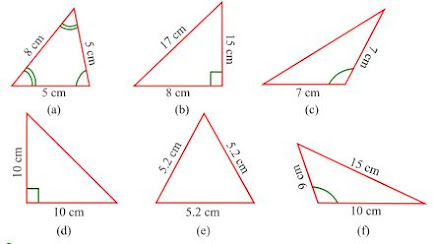Solution

(a) Acute angled triangle and Isosceles triangle

(b) Right-angled triangle and scalene triangle

(c) Obtuse-angled triangle and Isosceles triangle

(d) Right-angled triangle and Isosceles triangle

(e) Equilateral triangle and acute angled triangle

(f) Obtuse-angled triangle and scalene triangle

Question 4: Try to construct triangles using match sticks. Some are shown here.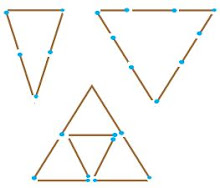Can you make a triangle with:

(a) 3 matchsticks?

(b) 4 matchsticks?

(c) 5 matchsticks?

(d) 6 matchsticks?

(Remember you have to use all the available matchsticks in each case)

If you cannot make a triangle, think of reasons for it.

Solution

(a) 3 matchsticks

This is an acute angle triangle and it is possible with 3 matchsticks to make a triangle because sum of two sides is greater than third side.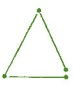(b) 4 matchsticks

This is a square, hence with four matchsticks we cannot make triangle.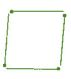(c) 5 matchsticks

This is an acute angle triangle and it is possible to make triangle with five matchsticks, in this case sum of two sides is greater than third side.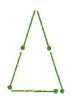(d) 6 matchsticks

This is an acute angle triangle and it is possible to make a triangle with the help of 6 matchsticks because sum of two sides is greater than third side.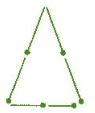Exercise 5.7

Question 1: Say true or false:

(a) Each angle of a rectangle is a right angle.

(b) The opposite sides of a rectangle are equal in length.

(c) The diagonals of a square are perpendicular to one another.

(d) All the sides of a rhombus are of equal length.

(e) All the sides of a parallelogram are of equal length.

(f) The opposite sides of a trapezium are parallel.

Solution

(a) True

(b) True

(c) True

(d) True

(e) False

(f) False

Question 2: Give reasons for the following:

(a) A square can be thought of as a special rectangle.

(b) A rectangle can be thought of as a special parallelogram.

(c) A square can be thought of as a special rhombus.

(d) Squares, rectangles, parallelograms are all quadrilateral.

(e) Square is also a parallelogram.

Solution

(a) Because its all angles are right angle and opposite sides are equal.

(b) Because its opposite sides are equal and parallel.

(c) Because its four sides are equal and diagonals are perpendicular to each other.

(d) Because all of them have four sides.

(e) Because its opposite sides are equal and parallel.

Question 3: A figure is said to be regular if its sides are equal in length and angles are equal in measure. Can you identify the regular quadrilateral?

Solution

A square is a regular quadrilateral.

Exercise 5.8

Question 1: Examine whether the following are polygons. If anyone among these is not, say why?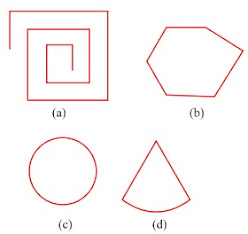Solution

(a) As it is not a closed figure, therefore, it is not a polygon.

(b) It is a polygon because it is closed by line segments.

(c) It is not a polygon because it is not made by line segments.

(d) It is not a polygon because it not made only by line segments, it has curved surface also.

Question 2: Name each polygon: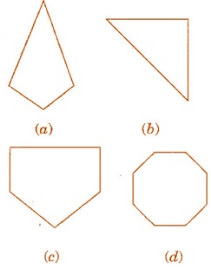Make two more examples of each of these.

Solution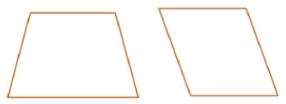(b) Triangle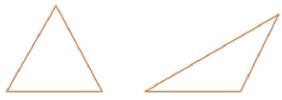(c) Pentagon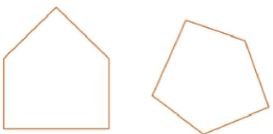(d) Octagon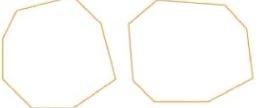Question 3: Draw a rough sketch of a regular hexagon. Connecting any three of its vertices, draw a triangle. Identify the type of the triangle you have drawn.

Solution

ABCDEF is a regular hexagon and triangle thus formed by joining AEF is an isosceles triangle.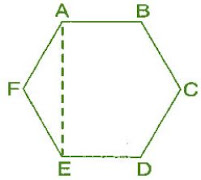Question 4: Draw a rough sketch of a regular hexagon. Connecting any three of its vertices, draw a triangle. Identify the type of the triangle you have drawn.

Solution

ABCDEFGH is a regular octagon and CDGH is a rectangle.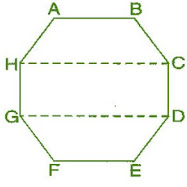Question 5: A diagonal is a line segment that joins any two vertices of the polygon and is not a side of the polygon. Draw a rough sketch of a pentagon and draw its diagonals.

Solution

ABCDE is the required pentagon and its diagonals are AD, AC, BE and BD.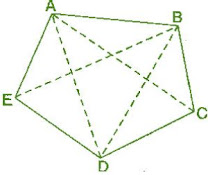Exercise 5.9

Question 1: Match the following: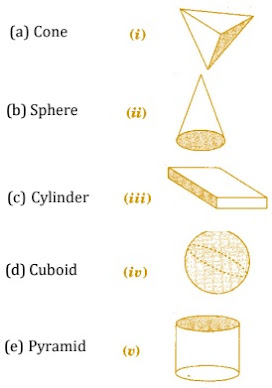Give two example of each shape.

Solution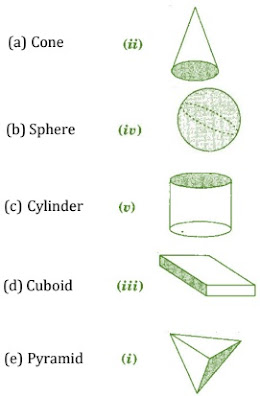Question 2: What shape is:

(b) A brick?

(c) A match box?